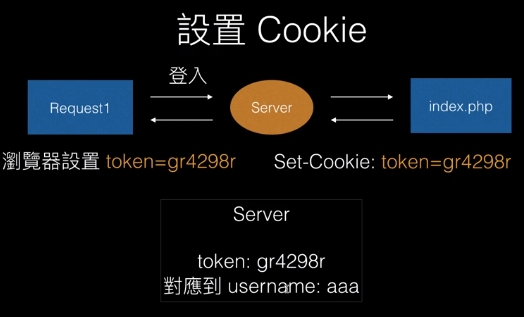# [BE101] 留言板(中-修正問題篇)

1. client 端資料都是可以改的，永遠抱持懷疑心態
2. 假設程式碼被偷，如何維持資料安全性 ?

# 修正問題：通行證機制簡介與實作## 第一步、建立 DB

// 實作一組隨機 16 碼的 token
function generateToken() {
$s = ''; for($i=1; $i<=16;$i++) {
$s .= chr(rand(65,90)); } return$s;
}
echo generateToken();


$token = generateToken();$sql = sprintf("INSERT INTO tokens(token, username) Values('%s', '%s')",
$token,$username
);
$result =$conn -> query($sql); if (!$result) {
die($conn -> error); }  在首頁，調整原本從 cookie 直接拿 username 的機制。變成先檢查 cookie 內有沒有 token，如果有將它拿出來，然後去資料庫查這個 token 對應到的 username。 $username = NULL;
if (!empty($_COOKIE['token'])) {$token = $_COOKIE['token'];$sql = sprintf(
"select username from tokens where token = '%s'",
$token );$result = $conn -> query($sql);
$row =$result -> fetch_assoc();
$username =$row['username'];
}


<?php
// 引入資料庫
require_once('conn.php');
// 刪除 token
$token =$_COOKIE['token'];
$sql = sprintf('DELETE FROM tokens WHERE token="%s"',$token);
$conn -> query($sql);
$expire = time() - 3600; setcookie("token", '',$expire);
?>


<?php
require_once('conn.php');

$username = NULL; if (!empty($_COOKIE['token'])) {
$token =$_COOKIE['token'];
$sql = sprintf( "select username from tokens where token = '%s'",$token
);
$result =$conn -> query($sql);$row = $result -> fetch_assoc();$username = $row['username']; }$result = $conn->query("SELECT * FROM comments ORDER BY id DESC"); if (!$result) {
die('錯誤訊息' . $conn->error); } ?>  調整新增留言的部分，原本從 cookie 抓 username 出來查，再去串他的 nickname。現在，要從 token 串 username，再從 username 串 nickname。這個流程很多地方都用的到，可以將他包成一個 funct。 要在 funct 中用 conn，要特別宣告為 global // utils.php function getUserFromToken($token) {
global $conn;$sql = sprintf(
"select username from tokens where token = '%s'",
$token );$result = $conn -> query($sql);
$row =$result -> fetch_assoc();
$username =$row['username'];

$sql = sprintf( "SELECT * FROM users WHERE username = '%s'",$username
);
$result =$conn -> query($sql);$row = $result -> fetch_assoc(); return$row; // username, id, nickname
}


// index.php
<?php
require_once('conn.php');
require_once('utils.php');
$username = NULL; if (!empty($_COOKIE['token'])) {
$user = getuserFromToken($_COOKIE['token']);
$username =$user['username'];
}

$result =$conn->query("SELECT * FROM comments ORDER BY id DESC");
if (!$result) { die('錯誤訊息' .$conn->error);
}
?>


// handle_add_comment.php
$user = getUserFromToken($_COOKIE['token']);
$nickname =$user['nickname'];


(上面的還不太熟)

# PHP 內建 session 機制

//handle_login.php
if ($result -> num_rows) {$_SESSION['username'] = \$username;
/*
1. 產生 session id(token)
*/